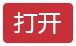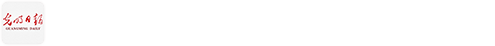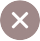﻿ <P>Clustering and Data Analysis</P> _推荐 _光明网
• 您想去哪里？
﻿

# Clustering and Data Analysis

2019-01-14 11:18　来源：留学杂志　丁立人
2019-01-14 11:18:46来源：留学杂志作者：丁立人责任编辑：王宏泽

1. Introduction

Clustering is a process of sorting objects, elements or data into groups according to their similarity or dissimilarity. In this thesis, topological foundation and several approaches are going to be explained.

2. Definition

In a set of data, a cluster is a group of elements in which the elements are more similar to each other than elements in other clusters. We can put these elements into a metric space to measure the similarity between them by a "distance". This function's purpose would be measure the similarity between two elements. Given a set X, a metric about X is a function X × X → R such that

1. d(x; y) ≥ 0 for all x; y ∈ X and d(x; y) = 0 i x = y.

2. d(x; y) = d(y; x) for all x; y ∈ X.

3. d(x; y) ≤ d(x; z) + d(z; y) for all x; y; z ∈ X.

A pair (X; d) is called a metric space. To form a cluster, we first define a relation x ∼R y as

x ∼R x′ iff d(x; x′) ≤ 2R

in which R ∈ R and R ≥ 0. This show these two element are similar. Then we can find a equivalence class accord to relation x ~R y defined with following: if there exists a sequence of elements x0, …… xn such that x = x0 ∼R x1, ……, xn−1 ∼R xn = y, then x ~R y.

Now set of equivalence classes about x forms a partition of the whole set, all elements in this class are more similar to each other comparing to elements not in the class--the cluster. Different functions aiming different type of data input. For data which can be quantify, they can be put into Rn then distance between two elements can be calculate. If data can't be quantified, then for C elements, a symmetric matrix C\C can be build and some function can be used to determine the similarity.

3. Clustering and data analysis

Clustering is one of the most vital task of data analysis, because clusters and process clusters form can indicate important information and underlying pattern which can't be provided by other methods.

4. Clustering algorithms

All clustering methods divide elements into groups in which elements are similar to each other using a similarity standard.

4.1 Hierarchical Clustering

Trying to form cluster, we would find that different threshold R form clusters with different size. If the threshold is 0, then the clusters would each only contain one element; As R increases, elements become connected and multiple clusters joined together and become one cluster. We can informally defines, that hierarchical clustering is the process finding such a hierarchy of clusters within a set of elements. We can use dendrograms shown hierarchy intuitively in (Figure 1.1), where each horizontal segment represent components being connected.

Bottom-up hierarchy is called an agglomerative clustering. We start from R = 0, when there are as many connected components as the number of individual points, as well as the number of clusters(Figure 1.2). As R increases, points start to become connected (Figure 1.3). At last, all elements in the data set are included in one cluster (Figure 1.1).

4.2 K-means Clustering

K-means Clustering is one of the most popular Flat Clustering algorithm. Unlike hierarchical clustering, flat clustering is focused on find the suitable R value.

4.3 Which one is better?

It's hard to say which method is better, since both of them have their advantage.

5. Clustering in Data Analysis Examples

The clustering data analysis example I use is the relation between GDP per capita and Fertility rate.

In our situation, there are some countries that have too few population so the data is missing. These data should be filtered out first. Since all data are in real number, we can map data into a Euclidean space. Many points locate near the x-axis, and some other near the fertility rate 2. This shows that there are many countries that have low GDP per capita have higher fertility rate, countries that have relatively higher GDP per capita have fertility rate around 2(Figure 3.1).

As the threshold increases, there are three clusters forming: cluster with F between 4 and 5, and with G under 5000\$; second one is located at the left-bottom corner of the graph, with fertility rate around 2 and G roughly around 10000\$; last one is the cluster with G from 30000\$ to 50000\$ and fertility rate around 2. In the first cluster, Congo rep, Ethiopia, Iraq, and South Sudan are suffer from poverty or war and have a high fertility rate with a low income level. The second cluster include countries such as China, Russia etc, rapidly growing recently. The third group are mostly consist of MDCs including UK, France, Canada etc. These countries are all highly developed and most of them have fertility rate less than two. Pattern of these three cluster actually is a strengthening evidence for the theory of demographic transition.

Figure 3.3 almost exactly give the partition of developing countries and developed countries.

6. Conclusion

Clustering is a very effective method in data analysis. I believe that the power of clustering is shown in the example about demo-graphics, in which clustering revealed three groups of countries that each on a different stage of demographic.

丁立人

年龄：17

城市：北京

年级：12

目标专业：数学，计算机科学

在夏校学习的一个月以来，我发现到应用拓扑学和之前初高中学的数学是完全不同的，应用拓扑和它的基础学科之一即线性代数对我来说是巨大的挑战。学习过程中给我留下印象最深的是聚簇算法，这是一种可以把有相似特征的数据归于几个相应的群中，还有空间变化，即通过函数将一个向量空间转化为另一个。从有所了解到能够写出这篇论文，我的进步绝不仅限于应用拓扑学相关的知识，还培养了独立研究的能力，并让我对高等数学更为严谨的逻辑有了一定的认识。

在论文中，我主要介绍了聚簇算法和拓扑的联系，以及用人口学相关的例子介绍了一种聚簇算法。[责任编辑:王宏泽][值班总编推荐]

[值班总编推荐]

[值班总编推荐]